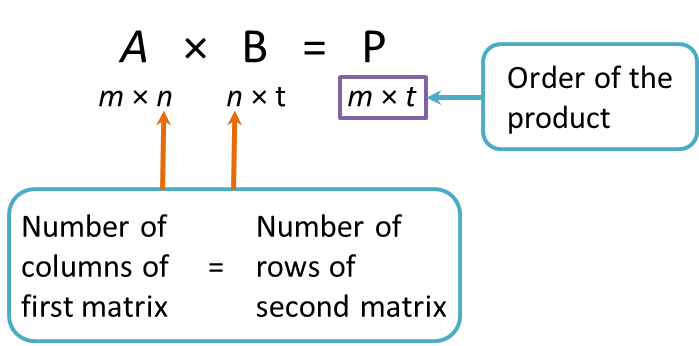2.5 Multiplication of Two Matrices

2.5 Multiplication of Two Matrices

1. Two matrices can be multiplied when the number of columns for the first matrix is the same as the number of rows for the second matrix.

For instance, if is a m × n matrix and B is a n × matrix, then the product of P = AB. The order of matrix P is m × tExamples:
Determine whether the following pairs of matrices can be multiplied and state the order of the product of those that can be multiplied.

Solution: